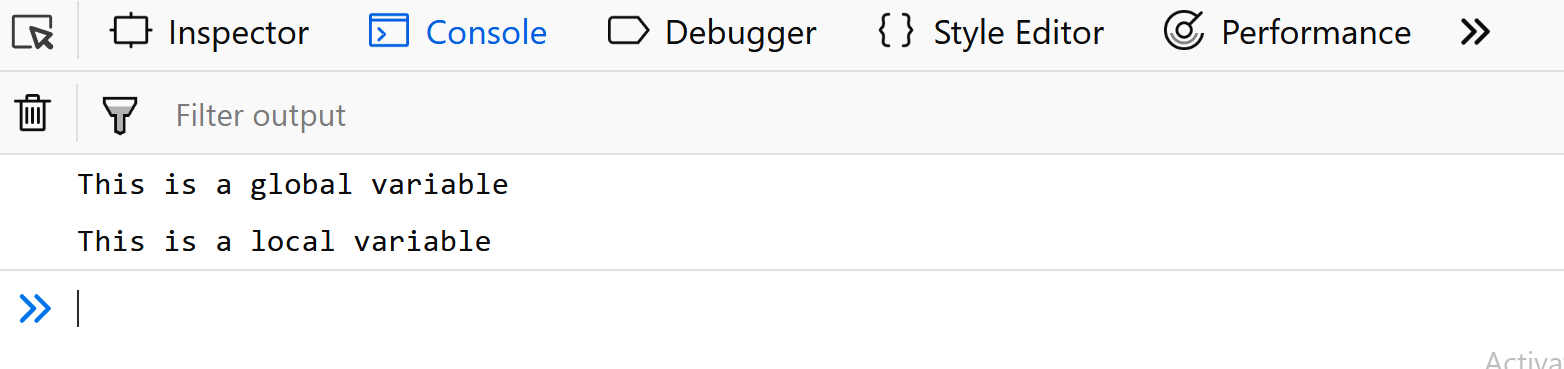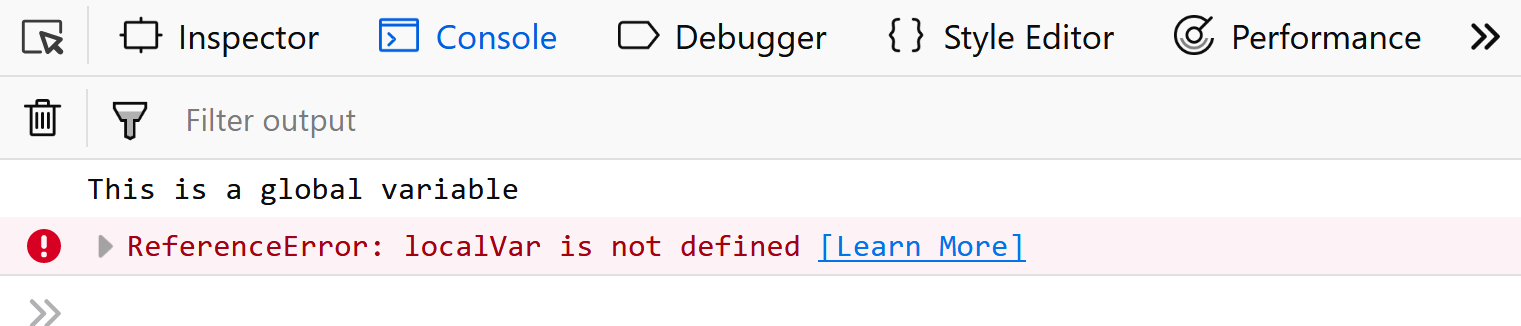# JavaScript Course | Variables in JavaScript

• Difficulty Level : Basic
• Last Updated : 14 Jun, 2022

Variables in JavaScript:

Variables in JavaScript are containers which hold reusable data. It is the basic unit of storage in a program.

• The value stored in a variable can be changed during program execution.
• A variable is only a name given to a memory location, all the operations done on the variable effects that memory location.
• In JavaScript, all the variables must be declared before they can be used.

Before ES2015, JavaScript variables were solely declared using the var keyword followed by the name of the variable and semi-colon. Below is the syntax to create variables in JavaScript:

```var var_name;
var x;```

The var_name is the name of the variable which should be defined by the user and should be unique. These type of names are also known as identifiers. The rules for creating an identifier in JavaScript are, the name of the identifier should not be any pre-defined word(known as keywords), the first character must be a letter, an underscore (_), or a dollar sign (\$). Subsequent characters may be any letter or digit or an underscore or dollar sign.
Notice in the above code sample, we didn’t assign any values to the variables.We are only saying they exist.If you were to look at the value of each variable in the above code sample, it would be undefined.
We can initialize the variables either at the time of declaration or also later when we want to use them. Below are some examples of declaring and initializing variables in JavaScript:

```// declaring single variable
var name;

// declaring multiple variables
var name, title, num;

// initializing variables
var name = "Harsh";
name = "Rakesh";```

Javascript is also known as untyped language. This means, that once a variable is created in javascript using the keyword var, we can store any type of value in this variable supported by javascript. Below is the example for this:

```// creating variable to store a number
var num = 5;

// store string in the variable num
num = "GeeksforGeeks";```

The above example executes well without any error in JavaScript unlike other programming languages.
Variables in JavaScript can also evaluate simple mathematical expressions and assume its value.

```// storing a mathematical expression
var x = 5 + 10 + 1;
console.log(x); // 16```

After ES2015, we now have two new variable containers : let and const. Now we shall look at both of them one by one. The variable type Let shares lots of similarities with var but unlike var it has scope constraints. To know more about them visit let vs var. Let’s make use of let variable:

```// let variable
let x; // undefined
let name = 'Mukul';

// can also declare multiple values
let a=1, b=2, c=3;

// assignment
let a = 3;
a = 4; // works same as var.```

Const is another variable type assigned to data whose value cannot and will not change throughout the script.

```// const variable
const name = 'Mukul';
name = 'Mayank'; // will give Assignment to constant variable error.```

Variable Scope in Javascript

Scope of a variable is the part of the program from where the variable may directly be accessible.
In JavaScript, there are two types of scopes:

1. Global Scope – Scope outside the outermost function attached to Window.
2. Local Scope – Inside the function being executed.

Let’s look at the code below. We have a global variable defined in first line in global scope. Then we have a local variable defined inside the function fun().

## JavaScript

 `let globalVar = ``"This is a global variable"``;` `function` `fun() {``  ``let localVar = ``"This is a local variable"``;` `  ``console.log(globalVar);``  ``console.log(localVar);``}` `fun();`

Output:When we execute the function fun(), the output shows that both global as well as local variables are accessible inside the function as we are able to console.log them. This shows that inside the function we have access to both global variables (declared outside the function) and local variables (declared inside the function).Let’s move the console.log statements outside the function and put them just after calling the function.

## JavaScript

 `let globalVar = ``"This is a global variable"``;` `function` `fun() {``  ``let localVar = ``"This is a local variable"``;``}` `fun();` `console.log(globalVar);``console.log(localVar);`

Output:We are still able to see the value of the global variable, but for local variable console.log throws an error. This is because now the console.log statements are present in global scope where they have access to global variables but cannot access the local variables.
Also, any variable defined in a function with the same name as a global variable takes precedence over the global variable, shadowing it.To understand variable scopes in details in JavaScript, please refer to the article on understanding variable scopes in Javascript.
Next Topic: JavaScript Course | Data Types in JavaScript

My Personal Notes arrow_drop_up